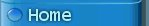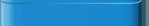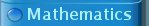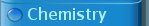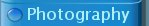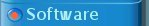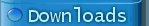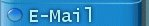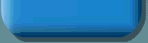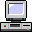### Miscellaneous useful utilities

Here, a set of small programs is presented, which can be very useful as part of other programs.

root finding and integration

Two very small, generic and quite fast root finding functions and a generic integration function, based on the so-called Romberg algorithm can be downloaded here. The file to be downloaded is numalg.tgz. The functions, which are given in this module are very robust and can be used as a workhorse for many quick implementations, where one needs to solve an equation or needs to compute an integral.

inverse laplace transformation

Especially, when one is working on electronics, then sometimes the tedious task of transforming a transform function in the laplace domain to a time-domain response needs to be performed. Here a simple program is presented, which can be used to perform an inverse laplace transform of any rational expression A(s) / B(s), with A(s) and B(s) being polynomials. First an initialization function must be called, with the coefficients of B(s) as argument and then for any A(s), given the B(s), an approximation of the inverse laplace transform can be computed easily. The file to be downloaded is laplace.tgz.

run-time expression parsing

A problem, which frequently occurs in C and C++ programs is that one wants to allow the parsing and evaluation of expressions, which are entered at run-time. This is a hard problem in a language like C or C++, because of the lack of language constructs, which allow run-time parsing of strings. This is exactly what this little program, presented here, does. It allows one to read a string from standard input and pass this as a mathematical expression to a function, which parses and evaluates this string. The string first is preparsed and then can be interpreted multiple times in a very fast way for different values of a set of variables. The file to be downloaded is parser.tgz.